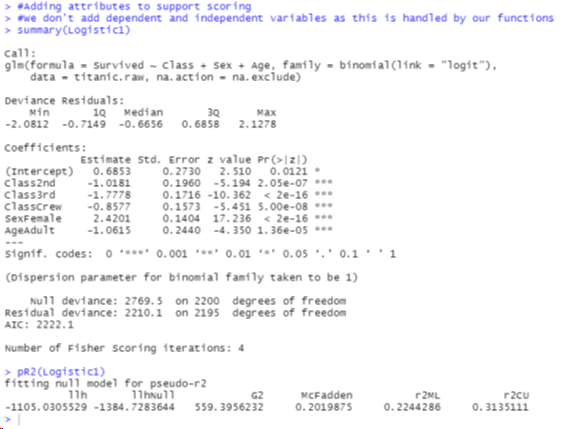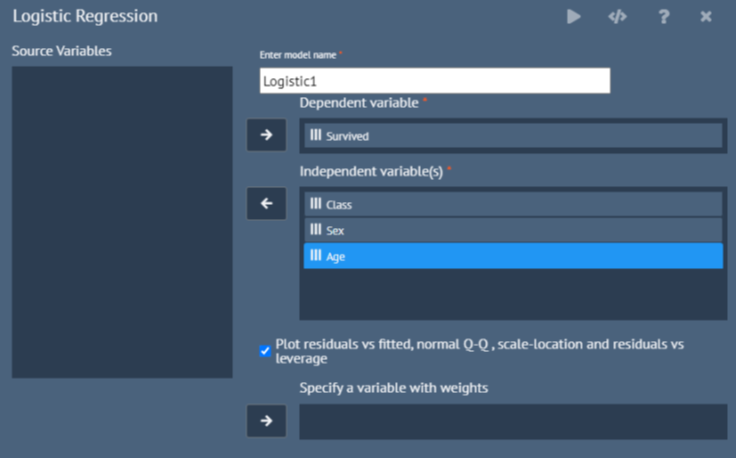## Prequisites

• You need to be on BlueSky Statistics 10.1
• You need very basic Javascript knowledge to create dialogs. Every dialog is a javascript file. There are several sample files that we provide and exercises to get you started.

## What Is A Dialog?

• GUI or a front end for making it easy to perform repeated analysis

Running a logistic model in R involves writing R code that does the following

• Creates the model
• Summarize the model
• Display the coefficients
• See the analysis of deviance
• Display pseudo rsquared statistics
• Display odds ratio
• See the formula
• Plot the residuals

There’s a lot of code to write

``````require(MASS);
require(pscl);
require(equatiomatic)
require(textutils)
#Builds a logistic model
Logistic1= glm(Survived ~ Class+Sex+Age, weights=, family =binomial(link='logit'), na.action=na.exclude,
data=titanic.raw)
#Display theoretical model equation and coefficients
#Display theoretical model
reg_formula = equatiomatic::extract_eq(Logistic1, raw_tex = FALSE,
wrap = TRUE, intercept = "alpha", ital_vars = FALSE)
BSkyFormat(reg_formula)
#Display coefficients
reg_equation = equatiomatic::extract_eq(Logistic1, use_coefs = TRUE,
wrap = TRUE,ital_vars = FALSE, coef_digits = BSkyGetDecimalDigitSetting() )
BSkyFormat(reg_equation)
#Summarizing the model
BSky_Logistic = summary(Logistic1)
#Analysis of variance
BSky_anova = anova(Logistic1, test="Chisq")
#odds ratio and 95% confidence interval
BSkyFormat(exp(cbind(OR=coef(Logistic1), confint.glm(Logistic1,level=0.95))),singleTableOutputHeader="Odds ratio(OR) and 95% Confidence interval ")
``````

The Output is not pretty• No code to write
• Eliminate 100’s -1000s lines of code
• Nicely formatted output
• Easy copy/paste into Microsoft Office apps
• Portable output
• Easy to save and distribute
• Reproducibility

## Sample Dialog

Use a point and click GUI instead of writing R code for popular analysis## Sample Output

See the nicely formatted output in tables that you can copy and paste into Microsoft Word, Excel…